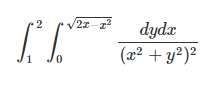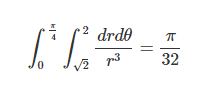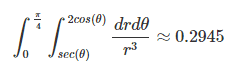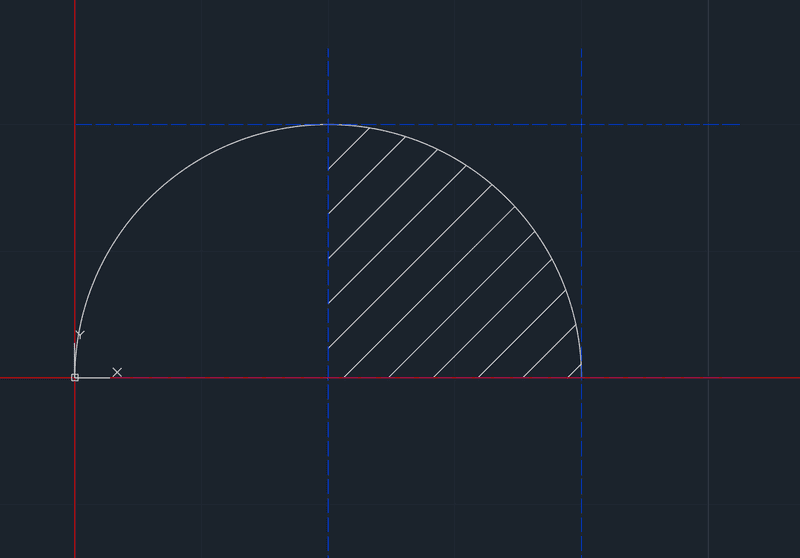# Stuck on the integration bound

Corey Williams

## Homework Statement

Trying to help a friend with a problem. We are supposed to solve the below using polar coordinates. The actual answer is supposed to be π/16. Solving the integral is not the issue, just converting it.2. The attempt at a solution
What I got sort of worked, but it is only half of the answer given.This is how I was reading the situation:
The hatch is the area that it seems we need to integrate over, and it also seems as though my answer shouldn't even be close given how it is a rectangular area.Is there something I'm not seeing, or is the provided answer wrong?

#### Attachments

Homework Helper
Gold Member
How did you get those integration limits of ##[\sqrt 2,2]## for r? ##r## is the distance from the origin to:
• the line ##y=1## for the lower limit and
• the circle boundary for the upper limit
Looking at the diagram, we see that, at ##\theta=0## the integration is for ##r\in[1,2]## and for ##\theta=\pi/4## it is for ##r\in [\sqrt 2,\sqrt 2]##. Those limits match the limits in the solution formula, but not the ones in the integral above it.

Corey Williams
From some of the examples I found you base r off of the origin to some point. From the origin to the top of the circle is a distance of √2. Having r ∈ [√2, 2cos(Θ)] Didn't work, but replacing it with its max at 2 made it a little bit closer to the answer.

Homework Helper
Gold Member
Draw a line segment from the origin, at an angle of ##\theta## above the x axis, to the point where it hits the curve.
The lower limit for the inner integral is the distance along that segment from the origin (call it O) to the point where the segment hits the vertical line ##x=1## (call that point Q).
The upper limit for the inner integral is the distance from O to where the line segment hits the curve.
Use geometry to calculate those two lengths as formulas in terms of ##\theta##.

Homework Helper
Dearly Missed

## Homework Statement

Trying to help a friend with a problem. We are supposed to solve the below using polar coordinates. The actual answer is supposed to be π/16. Solving the integral is not the issue, just converting it.
View attachment 215889

2. The attempt at a solution
What I got sort of worked, but it is only half of the answer given.
View attachment 215890

View attachment 215891

This is how I was reading the situation:
The hatch is the area that it seems we need to integrate over, and it also seems as though my answer shouldn't even be close given how it is a rectangular area.
View attachment 215892

Is there something I'm not seeing, or is the provided answer wrong?

Let the shaded shape in your diagram be ABCA, where AB and CA are horizontal and vertical line segments and BC is the circular arc. The origin is O.

One way to solve it using polar coordinates is to evaluate the integral over OBCO in polar coordinates, then subtract the integral over OACO.